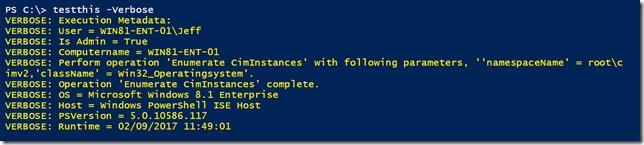# Doing More with PowerShell Verbose MessagesWhenever I teach about PowerShell scripting I always stress the value of using verbose messages in your functions and scripts. Assuming you are using cmdletbinding, and why wouldn’t you, you can insert Write-Verbose statements throughout your script. These statements won’t do anything unless your command is run with the common -Verbose parameter. However, these statements can be very useful for tracing and troubleshooting. I use these Verbose statements to help me track what my command is doing so that if it fails I have a better idea of where it failed and what it was doing. I don’t enjoy debugging and dealing with the interactive debugger and having Write-Verbose statements makes my life easier. Here are some ideas on how you might want to start using Write-Verbose.

First, if you want to try some of these things right from the command prompt, turn on your verbose pipeline.

```\$VerbosePreference = "continue"
```

When you are done testing, set it back to the default.

```\$VerbosePreference = "silentlycontinue"
```

Now you can run Write-Verbose commands to see what they look like. Remember that anything you want to write with Write-Verbose must be a string or at least be able to be treated as a string.

```Write-Verbose "I am doing something"
```

You may need to use subexpressions as well. One suggestion is to include a timestamp in your verbose message.

```Write-Verbose "[\$(Get-Date)] Starting to do something long running"
```
```Write-Verbose "\$((get-date).TimeOfDay.ToString()) Initializing array"
```

One thing that I tend to do in all my functions is use a verbose message to indicate what scriptblock is being processed.

```Function Get-Foo {
[cmdletbinding()]
Param(
[Parameter(Position = 0, Mandatory, ValueFromPipeline)]
[string[]]\$Computername
)
Begin {
Write-Verbose "[BEGIN  ] Starting: \$(\$MyInvocation.Mycommand)"
Write-Verbose "[BEGIN  ] Initializing foo array"
\$foo=@()
} #begin
Process {
foreach (\$computer in \$computername) {
Write-Verbose "[PROCESS] Getting foo from \$(\$computer.toUpper())"
#code happens
}
} #process
End {
Write-Verbose "[END    ] Ending: \$(\$MyInvocation.Mycommand)"
} #end
}
```

The Begin and End blocks include code to display the command name. This is very handy if you have a function that is calling another function. You may also be wondering about the spacing of END and BEGIN. I did that so that the verbose output would align nicely and be easier to read.

What about combining some of these things?

```Function TryMe {
[cmdletbinding()]
Param(
[string]\$Computername
)
Begin {
Write-Verbose "\$((get-date).TimeOfDay.ToString()) [BEGIN  ] Starting: \$(\$MyInvocation.Mycommand)"
Write-Verbose "\$((get-date).TimeOfDay.ToString()) [BEGIN  ] Initializing array"
\$a = @()
} #begin
Process {
Write-Verbose "\$((get-date).TimeOfDay.ToString()) [PROCESS] Processing \$Computername"
# code goes here
} #process
End {
Write-Verbose "\$((get-date).TimeOfDay.ToString()) [END    ] Ending: \$(\$MyInvocation.Mycommand)"
} #end
} #function
```

How about a variation that shows how much time is elapsing? Here’s a variation that displays a timespan calculated from the first command.

```Function TryMe2 {
[cmdletbinding()]
Param(
[Parameter(Position = 0, Mandatory,ValueFromPipeline)]
[string]\$Computername
)
Begin {
\$start = Get-Date
Write-Verbose "00:00:00.0000000 [BEGIN  ] Starting: \$(\$MyInvocation.Mycommand)"
Write-Verbose "\$((New-TimeSpan -Start \$start).ToString()) [BEGIN  ] Initializing array"
\$a = @()
} #begin
Process {
Write-Verbose "\$((New-TimeSpan -Start \$start).ToString()) [PROCESS] Processing \$Computername"
# code goes here
Start-Sleep -Milliseconds 1234
} #process
End {
Write-Verbose "\$((New-TimeSpan -Start \$start).ToString()) [END    ] Ending: \$(\$MyInvocation.Mycommand)"
} #end
} #function
```

One of the reasons I like to use Write-Verbose is that if someone is running one of my commands and encounters an error, I can have them start a transcript, which now works in the PowerShell ISE, run the command with -Verbose, stop the transcript, and send it to me. I can then see exactly what is happening (or not) for the user. With that in mind, I thought it might be helpful to include some verbose metadata.

```Function TestThis {
[cmdletbinding()]
Param()
\$id = [System.Security.Principal.WindowsIdentity]::GetCurrent()
Write-Verbose "Computername = \$env:COMPUTERNAME"
Write-Verbose "OS = \$((Get-CimInstance Win32_Operatingsystem).Caption)"
Write-Verbose "Host = \$(\$host.Name)"
Write-Verbose "PSVersion = \$(\$PSVersionTable.PSVersion)"
Write-Verbose "Runtime = \$(Get-Date)"
}```

That might be a bit much to include in every script. What could be easier is an external function I could load in my profile.

```Function Get-ExecutionMetadata {
[cmdletbinding()]
Param()
\$id = [System.Security.Principal.WindowsIdentity]::GetCurrent()
\$os = (Get-CimInstance Win32_Operatingsystem).Caption
\$meta = [pscustomobject]@{
Computername = \$env:COMPUTERNAME
OS = \$os
Host = \$(\$host.Name)
PSVersion = \$(\$PSVersionTable.PSVersion)
Runtime = \$(Get-Date)
}
\$meta
}
```

I could then call this code in my function.

```Function Get-Foo {
[cmdletbinding()]
Param(
[Parameter(Position = 0, Mandatory, ValueFromPipeline)]
[string[]]\$Computername
)
Begin {
Write-Verbose "[BEGIN  ] Starting: \$(\$MyInvocation.Mycommand)"
#don't pass -Verbose to the Get-ExecutionMetadata function
Write-Verbose "[BEGIN  ] Initializing foo array"
\$foo=@()
} #begin
Process {
foreach (\$computer in \$computername) {
Write-Verbose "[PROCESS] Getting foo from \$(\$computer.toUpper())"
#code happens
[pscustomobject]@{Computername = \$computer;Time = (Get-Date).TimeOfDay}
}
} #process
End {
Write-Verbose "[END    ] Ending: \$(\$MyInvocation.Mycommand)"
} #end
}
```